# Addition of Three 3-digit numbers Ones to Tens | Maths Grade 2

### Addition of Three 3-digit numbers with Regrouping Ones to Tens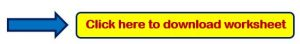Example 1:- Add the following?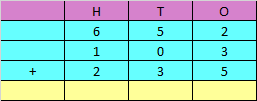Explanation:

Step 1: Adding the digits at one’s place,

2 + 3 + 5 = 10

Since the number is more than 9, we need to carry over 1 to the tens place.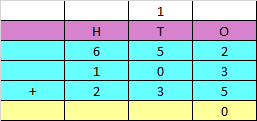Step 2: Adding the digits at tens place and the carry of 1,

5 + 0 + 3 + 1 = 9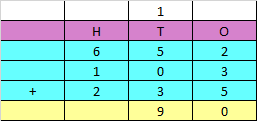Step 3: Adding the digits at hundreds place,

6 + 1 + 2 = 9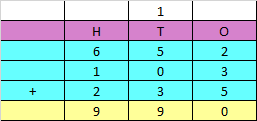Hence, the sum of the given numbers is 990

Example 2:- Add the following?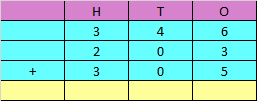Explanation:

Step 1: Adding the digits at one’s place,

6 + 3 + 5 = 14

Since the number is more than 9, we need to carry over 1 to the tens place.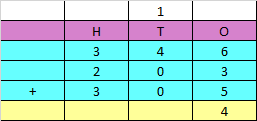Step 2: Adding the digits at tens place and the carry of 1,

4 + 0 + 0 + 1 = 5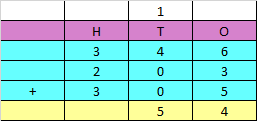Step 3: Adding the digits at hundreds place,

3 + 2 + 3 = 8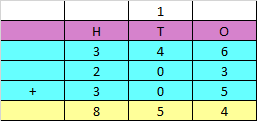Hence, the sum of the given numbers is 854

Example 3:- Add the following?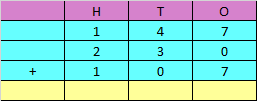Explanation:

Step 1: Adding the digits at one’s place,

7 + 0 + 7 = 14

Since the number is more than 9, we need to carry over 1 to the tens place.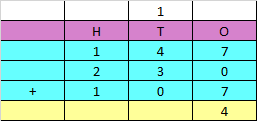Step 2: Adding the digits at tens place and the carry of 1,

4 + 3 + 0 + 1 = 8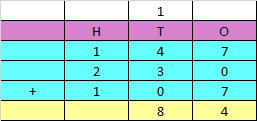Step 3: Adding the digits at hundreds place,

1 + 2 + 1 = 4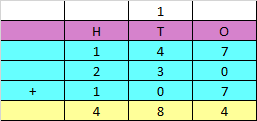Hence, the sum of the given numbers is 484